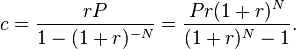# Everyday Calculation

Free calculators and unit converters for general and everyday use.

Calculators » Finance » Loan Affordability

## Loan Amount Calculator

Our online tools will provide quick answers to your calculation and conversion needs. On this page, you can calculate loan affordability i.e., Find the loan amount you can borrow by entering the monthly repayment amount you are comfortable with.

% p.a.

Result window

Download: Use this loan calculator offline with our all-in-one calculator app for Android and iOS.

To calculate loan amount where you make repayments in daily, quarterly or yearly installments, use the present value of annuity calculator. Select "Solve for Amount" and enter the repayment amount in the "periodic payment amount" field.

#### Loan payoff calculation

The loan amount can be calculated by solving for P in the mortgage formula shown below:Where,
c = periodic payment,
r = loan interest rate per month in % (annual interest rate in % divided by 12)
N = loan term in months

For calculating savings on early loan repayment, click on the calculator linked below.